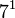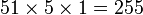# Groups of order 6048

## Contents

See pages on algebraic structures of order 6048| See pages on groups of a particular order

## Statistics at a glance

### Factorization and useful forms

The number 6048 has the following factorization with prime factors 2,3,7:$6048 = 2^5 \cdot 3^3 \cdot 7^1 = 32 \cdot 27 \cdot 7$

### Group counts

All groups of this order have not yet been classified. The information below is therefore partial.

Quantity Value Explanation
Total number of groups up to isomorphism unknown
Number of abelian groups (i.e., finite abelian groups) up to isomorphism 21 (Number of abelian groups of order$2^5$) times (Number of abelian groups of order$3^3$) times (Number of abelian groups of order$7^1$)= (number of unordered integer partitions of 5) times (number of unordered integer partitions of 3) times (number of unordered integer partitions of 1) =$7 \times 3 \times 1= 21$. See classification of finite abelian groups and structure theorem for finitely generated abelian groups.
Number of nilpotent groups (i.e., finite nilpotent groups) up to isomorphism 255 (Number of groups of order 32) times (Number of groups of order 27) times (Number of groups of order 7)=$51 \times 5 \times 1 = 255$. See number of nilpotent groups equals product of number of groups of order each maximal prime power divisor, which in turn follows from equivalence of definitions of finite nilpotent group.
Number of solvable groups (i.e., finite solvable groups) up to isomorphism unknown
Number of simple groups up to isomorphism (since the number is composite, this equals the number of simple non-abelian groups) 1 projective special unitary group:PSU(3,3)
Number of almost simple groups up to isomorphism 1 same as the simple group
Number of quasisimple groups up to isomorphism 1 same as the simple group
Number of almost quasisimple groups up to isomorphism 1 same as the simple group
Number of semisimple groups up to isomorphism 1 same as the simple group
Number of perfect groups up to isomorphism 1 same as the simple group